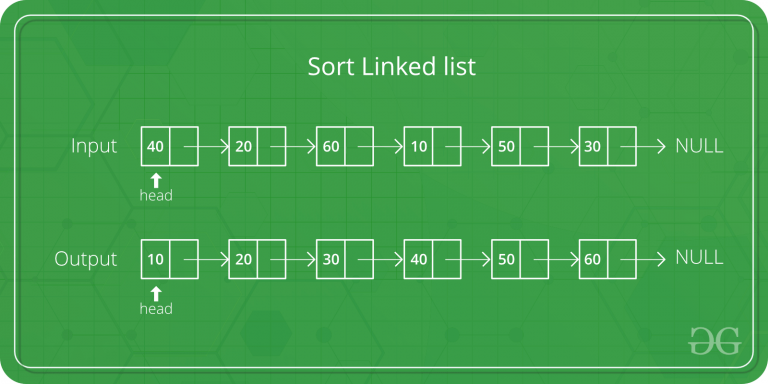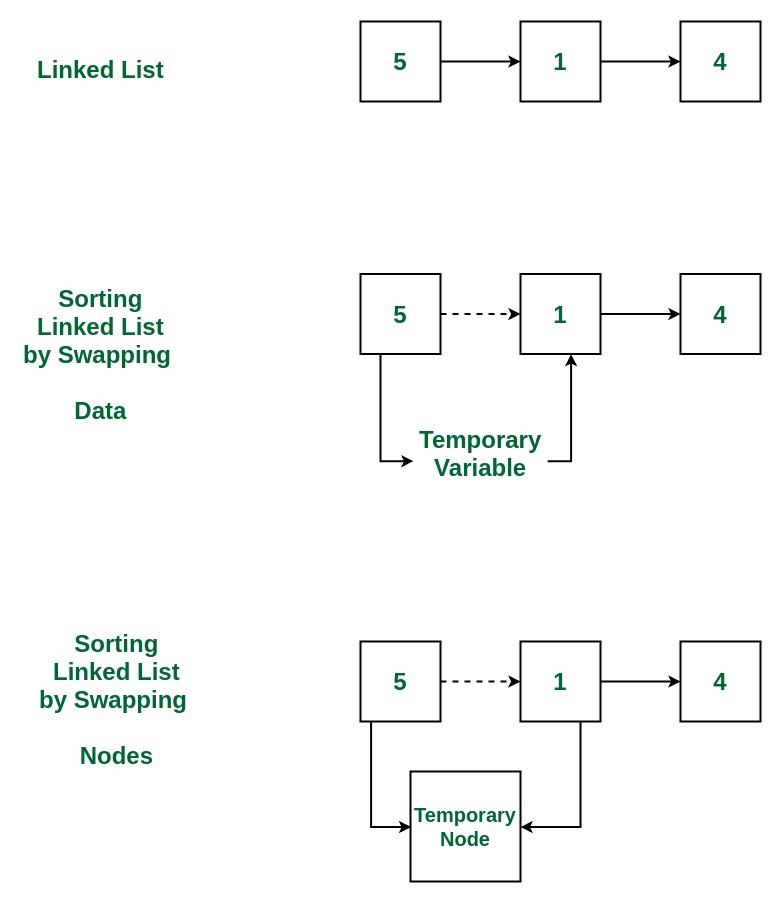Skip to content
Related Articles
Bubble Sort for Linked List by Swapping nodes
• Difficulty Level : Easy
• Last Updated : 04 Mar, 2020

Given a singly linked list, sort it using bubble sort by swapping nodes.Example:

```Input: 10->30->20->5
Output: 5->10->20->30

Input: 20->4->3
Output: 3->4->20```## Recommended: Please try your approach on {IDE} first, before moving on to the solution.

Approach:

1. Get the Linked List to be sorted
2. Apply Bubble Sort to this linked list, in which, while comparing the two adjacent nodes, actual nodes are swapped instead of just swapping the data.
3. Print the sorted list

Below is the implementation of the above approach:

## C++

 `// C++ program to sort Linked List``// using Bubble Sort``// by swapping nodes`` ` `#include ``using` `namespace` `std;`` ` `/* structure for a node */``struct` `Node ``{``    ``int` `data;``    ``struct` `Node* next;``} Node;`` ` `/*Function to swap the nodes */``struct` `Node* swap(``struct` `Node* ptr1, ``struct` `Node* ptr2)``{``    ``struct` `Node* tmp = ptr2->next;``    ``ptr2->next = ptr1;``    ``ptr1->next = tmp;``    ``return` `ptr2;``}`` ` `/* Function to sort the list */``int` `bubbleSort(``struct` `Node** head, ``int` `count)``{``    ``struct` `Node** h;``    ``int` `i, j, swapped;`` ` `    ``for` `(i = 0; i <= count; i++)``    ``{`` ` `        ``h = head;``        ``swapped = 0;`` ` `        ``for` `(j = 0; j < count - i - 1; j++) ``        ``{`` ` `            ``struct` `Node* p1 = *h;``            ``struct` `Node* p2 = p1->next;`` ` `            ``if` `(p1->data > p2->data)``            ``{`` ` `                ``/* update the link after swapping */``                ``*h = swap(p1, p2);``                ``swapped = 1;``            ``}`` ` `            ``h = &(*h)->next;``        ``}`` ` `        ``/* break if the loop ended without any swap */``        ``if` `(swapped == 0)``            ``break``;``    ``}``}`` ` `/* Function to print the list */``void` `printList(``struct` `Node* n)``{``    ``while` `(n != NULL)``    ``{``        ``cout << n->data << ``" -> "``;``        ``n = n->next;``    ``}``    ``cout << endl;``}`` ` `/* Function to insert a struct Node``at the beginning of a linked list */``void` `insertAtTheBegin(``struct` `Node** start_ref, ``int` `data)``{``    ``struct` `Node* ptr1``        ``= (``struct` `Node*)``malloc``(``sizeof``(``struct` `Node));`` ` `    ``ptr1->data = data;``    ``ptr1->next = *start_ref;``    ``*start_ref = ptr1;``}`` ` `// Driver Code``int` `main()``{``    ``int` `arr[] = { 78, 20, 10, 32, 1, 5 };``    ``int` `list_size, i;`` ` `    ``/* start with empty linked list */``    ``struct` `Node* start = NULL;``    ``list_size = ``sizeof``(arr) / ``sizeof``(arr);`` ` `    ``/* Create linked list from the array arr[] */``    ``for` `(i = 0; i < list_size; i++)``        ``insertAtTheBegin(&start, arr[i]);`` ` `    ``/* print list before sorting */``    ``cout <<``"Linked list before sorting\n"``;``    ``printList(start);`` ` `    ``/* sort the linked list */``    ``bubbleSort(&start, list_size);`` ` `    ``/* print list after sorting */``    ``cout <<``"Linked list after sorting\n"``;``    ``printList(start);`` ` `    ``return` `0;``}`` ` `// This code is contributed by``// shubhamsingh10`

## C

 `// C program to sort Linked List``// using Bubble Sort``// by swapping nodes`` ` `#include ``#include `` ` `/* structure for a node */``struct` `Node {``    ``int` `data;``    ``struct` `Node* next;``} Node;`` ` `/*Function to swap the nodes */``struct` `Node* swap(``struct` `Node* ptr1, ``struct` `Node* ptr2)``{``    ``struct` `Node* tmp = ptr2->next;``    ``ptr2->next = ptr1;``    ``ptr1->next = tmp;``    ``return` `ptr2;``}`` ` `/* Function to sort the list */``int` `bubbleSort(``struct` `Node** head, ``int` `count)``{``    ``struct` `Node** h;``    ``int` `i, j, swapped;`` ` `    ``for` `(i = 0; i <= count; i++) {`` ` `        ``h = head;``        ``swapped = 0;`` ` `        ``for` `(j = 0; j < count - i - 1; j++) {`` ` `            ``struct` `Node* p1 = *h;``            ``struct` `Node* p2 = p1->next;`` ` `            ``if` `(p1->data > p2->data) {`` ` `                ``/* update the link after swapping */``                ``*h = swap(p1, p2);``                ``swapped = 1;``            ``}`` ` `            ``h = &(*h)->next;``        ``}`` ` `        ``/* break if the loop ended without any swap */``        ``if` `(swapped == 0)``            ``break``;``    ``}``}`` ` `/* Function to print the list */``void` `printList(``struct` `Node* n)``{``    ``while` `(n != NULL) {``        ``printf``(``"%d -> "``, n->data);``        ``n = n->next;``    ``}``    ``printf``(``"\n"``);``}`` ` `/* Function to insert a struct Node``   ``at the beginning of a linked list */``void` `insertAtTheBegin(``struct` `Node** start_ref, ``int` `data)``{``    ``struct` `Node* ptr1``        ``= (``struct` `Node*)``malloc``(``sizeof``(``struct` `Node));`` ` `    ``ptr1->data = data;``    ``ptr1->next = *start_ref;``    ``*start_ref = ptr1;``}`` ` `// Driver Code``int` `main()``{``    ``int` `arr[] = { 78, 20, 10, 32, 1, 5 };``    ``int` `list_size, i;`` ` `    ``/* start with empty linked list */``    ``struct` `Node* start = NULL;``    ``list_size = ``sizeof``(arr) / ``sizeof``(arr);`` ` `    ``/* Create linked list from the array arr[] */``    ``for` `(i = 0; i < list_size; i++)``        ``insertAtTheBegin(&start, arr[i]);`` ` `    ``/* print list before sorting */``    ``printf``(``"Linked list before sorting\n"``);``    ``printList(start);`` ` `    ``/* sort the linked list */``    ``bubbleSort(&start, list_size);`` ` `    ``/* print list after sorting */``    ``printf``(``"Linked list after sorting\n"``);``    ``printList(start);`` ` `    ``return` `0;``}`
Output:
```Linked list before sorting
5 -> 1 -> 32 -> 10 -> 20 -> 78 ->
Linked list after sorting
1 -> 5 -> 10 -> 20 -> 32 -> 78 ->
```

Attention reader! Don’t stop learning now. Get hold of all the important DSA concepts with the DSA Self Paced Course at a student-friendly price and become industry ready.  To complete your preparation from learning a language to DS Algo and many more,  please refer Complete Interview Preparation Course.

In case you wish to attend live classes with industry experts, please refer Geeks Classes Live

My Personal Notes arrow_drop_up